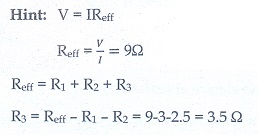# Unit II: Current Electricity - Online Test

Q1. The following graph shows current versus voltage values of some unknown conductor. What is the resistance of this conductor?Explaination / Solution:Q2. A wire of resistance 2 ohms per meter is bent to form a circle of radius 1m. The equivalent resistance between its two diametrically opposite points, A and B as shown in the figure is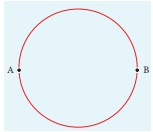Explaination / Solution:Q3. A toaster operating at 240 V has a resistance of 120 Ω. The power is
Explaination / Solution: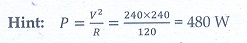Q4. A carbon resistor of (47 ± 4.7 ) k Ω to be marked with rings of different colours for its identification. The colour code sequence will be
Explaination / Solution: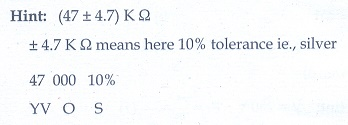Q5. What is the value of resistance of the following resistor?Explaination / Solution: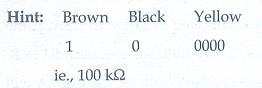Q6. Two wires of A and B with circular cross section made up of the same material with equal lengths. Suppose RA = 3 RB, then what is the ratio of radius of wire A to that of B?
Explaination / Solution:Q7. A wire connected to a power supply of 230 V has power dissipation P1. Suppose the wire is cut into two equal pieces and connected parallel to the same power supply. In this case power dissipation is P2. The ratio P2 / P1 is
Explaination / Solution:Q8. In India electricity is supplied for domestic use at 220 V. It is supplied at 110 V in USA. If the resistance of a 60W bulb for use in India is R, the resistance of a 60W bulb for use in USA will be
Explaination / Solution:Q9. In a large building, there are 15 bulbs of 40W, 5 bulbs of 100W, 5 fans of 80W and 1 heater of 1kW are connected. The voltage of electric mains is 220V. The minimum capacity of the main fuse of the building will be
Explaination / Solution: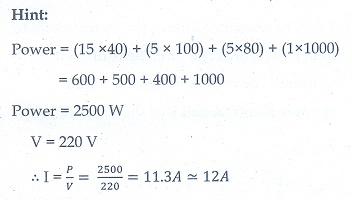Q10. There is a current of 1.0 A in the circuit shown below. What is the resistance of P ?Explaination / Solution: